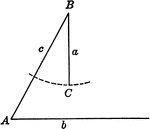### Ambiguous Case of Law of Sines Triangle

Illustration showing ambiguous case when the solution is not a triangle using law of sines.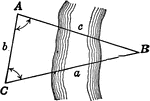### Oblique Triangle for Distance Across a River

Illustration of oblique triangle used to find distance across a river.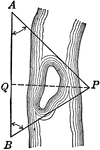### Oblique Triangle for Distance Across a River

Illustration of oblique triangle used to find distance across a river.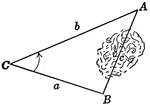### Oblique Triangle for Distance Across Lake

Illustration of oblique triangle used to find distance across a lake.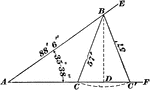### Oblique Triangle Used For Ambiguous Case

Oblique triangle with perpendicular drawn and angle of measure 35 ° 38" given, and sides of 88'…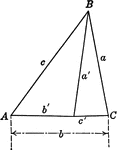### Triangles ABC and ABC'

Triangle ABC and triangle ABC'. This illustration could be used to demonstrate the law of sines.